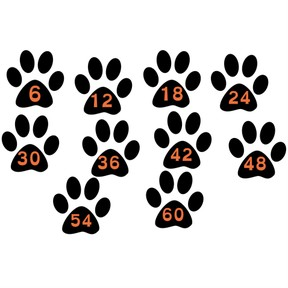Solving the 6 times table

# Solving the 6 times table

I can solve the times 6 multiplication facts.

No account needed.8,000 schools use Gynzy92,000 teachers use Gynzy1,600,000 students use Gynzy

## General

Students will learn multiplication strategies for the times 6 facts. Instruction and practice will cover skip counting and multiples along with using the times 5 facts and adding more set.

## Standards

CCSS.Math.Content.3.OA.B.5

## Learning objective

Students will be able to solve times 6 multiplication facts.

## Introduction

Students will reflect on facts and strategies they have learned. They will practice a set of facts with The Gynzy Math Problem Generator.

## Instruction

Students will begin by thinking about the strategies they already have used to learn the 0 - 5multiplication facts. Students review the identity, zero, and commutative properties of multiplication. Next, students practice skip-counting the multiples of 6, and practice is provided. Students then review the times 5 facts and use the strategy of times 5 plus one more group to solve ×6 equations. Students will demonstrate the skill by drawing the additional group. Students then practice the facts again by spinning a wheel that matches a color to reveal a fact on a dartboard. The lesson concludes with two word problems using the times 6 facts.

## Quiz

Students respond to ten multiple-choice and true/false questions.

## Closing

Students outline arrays on graph paper. These are colored and decorated. The idea of tessellation is discussed.

## Instruction materials

- graph paper
- colored pencils, crayons, or markers (something to color with)

### The online teaching platform for interactive whiteboards and displays in schools

• Save time building lessons

• Manage the classroom more efficiently

• Increase student engagement